#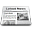Using pROC

Now that pROC is released, it is time to see how it can be used. Here is an example of a typical analysis: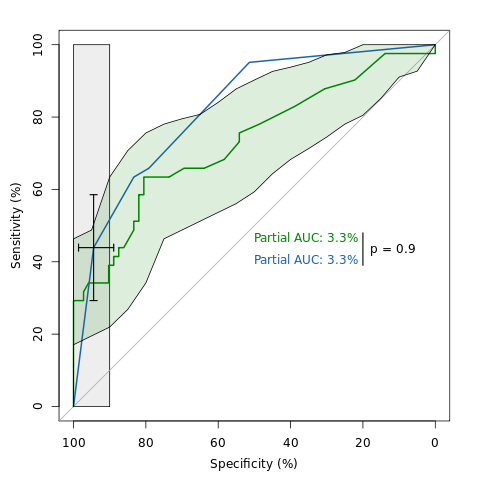An example of ROC analysis with pROC.

Here is the code that was used to generate this image. The first step is to load pROC (don't forget to type `install.packages("pROC")` if you haven't installed pROC yet!) and the aSAH data that is provided:

```library(pROC)
data(aSAH)```

First we'll build the ROC curves. We want the sensitivity and specificity in % (thus `percent = TRUE`) and a partial AUC between 90 and 100% specificity:

```rocs100 <- roc(aSAH\$outcome, aSAH\$s100, percent = TRUE, partial.auc=c(100, 90))
rocwfns <- roc(aSAH\$outcome, aSAH\$wfns, percent = TRUE, partial.auc=c(100, 90))```

Then we compute confidence intervals. For S100, we compute the confidence of sensitivity over several levels of specificity. This will allow us to plot the interval as a shape. With WFNS, we compute the confidence interval of the cut-off 4.5. We use 10000 bootstrap replicates to get a good estimation:

```cis100 <- ci(rocs100, of = "se", sp = seq(0, 100, 5), boot.n=10000)
ciwfns <- ci(rocwfns, of = "threshold", threshold=4.5, boot.n=10000)```

Now, the main feature of pROC is the comparison of two ROC curves. Again we use 10000 bootstrap replicates to get a good estimation:

`rtest <- roc.test(rocs100, rocwfns, boot.n=10000)`

And finally we plot the ROC curves:

```png("demo.png")
plot(rocs100, col="#008600", max.auc.polygon=TRUE, print.auc=TRUE, print.auc.y=48)

And the confidence intervals:

```plot(cis100, type="shape", col="#00860022", no.roc=TRUE)
plot(ciwfns, type="bars", lwd=1.5)```

Last we add the p value of the ROC comparison test:

```text(18, 45, sprintf("p = %.1f", rtest\$p.value), adj=c(0,1))
lines(c(20, 20), c(39, 48), lwd=1.5)
dev.off()```

And that's it, we have generated the image above!

You can find more such examples on the screenshots page on ExPASy!

Xavier Robin
Published Tuesday, April 27, 2010 20:50 CEST
Tags: pROC

#pROC 1.0 released

After years of work with ROC curves in R, first with packages such as ROCR and verification, then with custom functions; and 4 months of development to create a coherent package, extensive discussions about its name (SwissROC was the first thought (but is Switzerland still a selling argument?), or Rocker (that's a bit similar to the already-existing ROCR)) and its licensing (let's go with GPL), I'm glad to announce the release of pROC!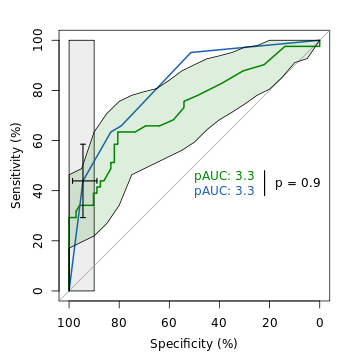An example of ROC analysis with pROC.

pROC is a set of tools to visualize, smooth and compare receiver operating characteristic (ROC curves). Full or partial area under the curve (AUC) can be compared with statistical tests based on U-statistics or bootstrap. Confidence intervals can be computed for (p)AUC or ROC curves. It is available for R (command-line interface) and S+ (with an additional graphical user interface).

You can find it on ExPASy (thanks Céline from the SIB web-team) and in a few minutes on the CRAN (it has to pass a few checks before it is formally accepted).

But there is no need to download the package. The installation can be done in one command directly from R:

`install.packages("pROC")`

The package must then be loaded with:

`library(pROC)`

To get help, enter the following in the R prompt:

`?pROC`

And that's it! You can have a look at the screenshots to see what it looks like.

Simultaneously we submitted a paper (a short application note) describing it to Bioinformatics. Crossed fingers in the hope it will be accepted!

2010-06-15 follow-up: the paper has been rejected. We will resubmit it to BMC Bioinformatics.

2011-03-17 follow-up: the paper was published in BMC Bioinformatics.

Xavier Robin
Published Tuesday, April 27, 2010 20:42 CEST
Tags: pROC

##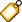Tags

Background noise Books Computers Fun Hobbies Internet Me Mozilla My website Photo Politics Programming School Software Ubuntu pROC

##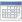Calendar

MonTueWedThuFriSatSun
1234
567891011
12131415161718
19202122232425
2627282930